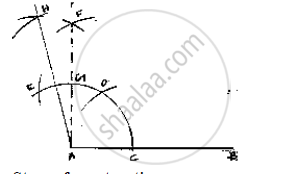# Construct the Angle of the Measurement: 1 . 105° - Mathematics

Construct the angle of the measurement:

1 . 105°

#### SolutionSteps of construction:
1. Draw a line segment AB.
2. With center A and any radius, draw an arc which intersect AB at C.
3. With center C and same radius, draw an arc which intersects previous arc at D.
4. With center D and same radius, draw an arc which interest are in (2) at E
5. With centers E and D and radius more than 1/2ED,  draw arcs intersecting each other at F.
6. Join FA which intersects arc in (2) at E
7. With centers E and G, and radius more than half of EG, draw arcs intersecting eachother at H.
8. Join HA
∠HAB =105°

Concept: Basic Constructions
Is there an error in this question or solution?

#### APPEARS IN

RD Sharma Mathematics for Class 9
Chapter 16 Constructions
Exercise 16.2 | Q 11.3 | Page 9

Share